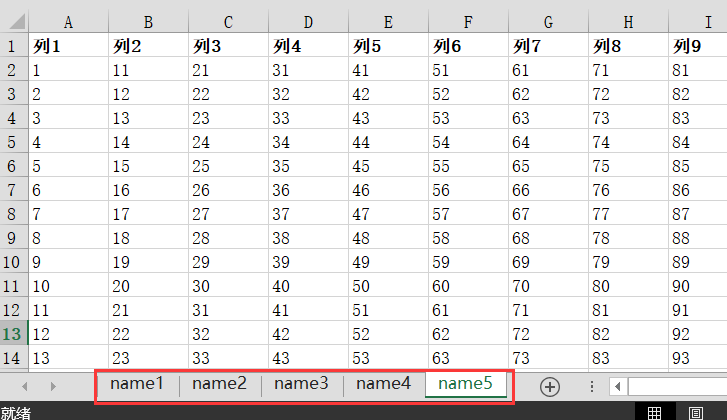# 【数据处理】pandas DataFrame 对多个sheet表格的excel 读取、处理、保存### 生成文件列表和sheet名称列表

import pandas as pd
import os

# 将excel中的sheet名称放入列表
sheet_names = ["name1","name2","name3","name4", ... ]
# 将excel文件名称放入列表
xlsx_names = [x for x in os.listdir() if x.endswith(".xlsx")]


## 循环读取并保存

writer = pd.ExcelWriter('result.xlsx',engine='openpyxl')


num = 1
for sheet_name in sheet_names:
df = None
for xlsx_name in xlsx_names:
_df = pd.read_excel(xlsx_name, sheet_name=sheet_name)
if df is None:
df = _df
else:
df = pd.concat([df, _df], ignore_index=True)
# 下面的保存文件处填写writer，结果会不断地新增sheet，避免循环时被覆盖
df.to_excel(excel_writer=writer, sheet_name=sheet_name, encoding="utf-8", index=False)
print(sheet_name + "  保存成功！共%d个，第%d个。" % (len(sheet_names),num))
num += 1
writer.save()
writer.close()